Related Articles
Multithreading in Python | Set 1
• Difficulty Level : Easy
• Last Updated : 01 Apr, 2020

This article covers the basics of multithreading in Python programming language. Just like multiprocessing, multithreading is a way of achieving multitasking. In multithreading, the concept of threads is used.

Let us first understand the concept of thread in computer architecture.

Thread

In computing, a process is an instance of a computer program that is being executed. Any process has 3 basic components:

• An executable program.
• The associated data needed by the program (variables, work space, buffers, etc.)
• The execution context of the program (State of process)

A thread is an entity within a process that can be scheduled for execution. Also, it is the smallest unit of processing that can be performed in an OS (Operating System).

In simple words, a thread is a sequence of such instructions within a program that can be executed independently of other code. For simplicity, you can assume that a thread is simply a subset of a process!

A thread contains all this information in a Thread Control Block (TCB):

• Thread Identifier: Unique id (TID) is assigned to every new thread
• Stack pointer: Points to thread’s stack in the process. Stack contains the local variables under thread’s scope.
• Program counter: a register which stores the address of the instruction currently being executed by thread.
• Thread state: can be running, ready, waiting, start or done.
• Thread’s register set: registers assigned to thread for computations.
• Parent process Pointer: A pointer to the Process control block (PCB) of the process that the thread lives on.

Consider the diagram below to understand the relation between process and its thread: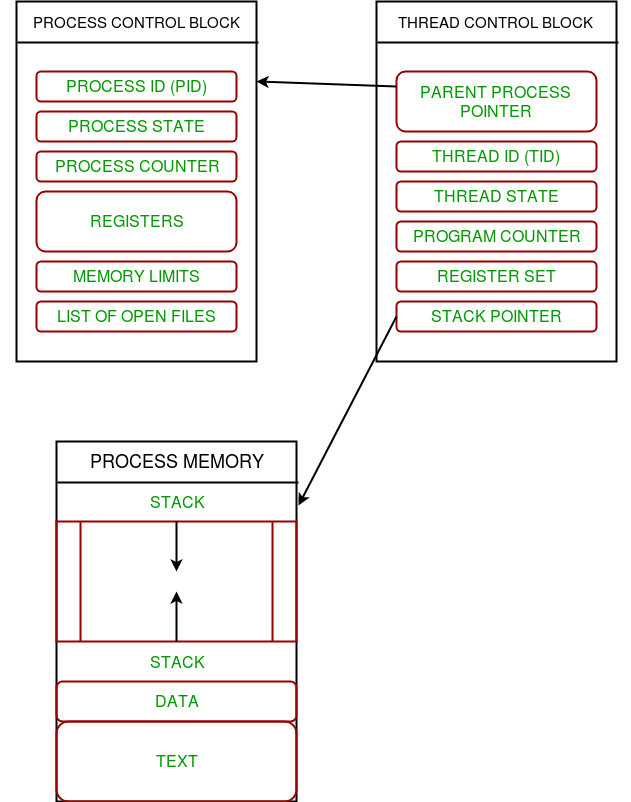Multithreading

Multiple threads can exist within one process where:

• Each thread contains its own register set and local variables (stored in stack).
• All thread of a process share global variables (stored in heap) and the program code.

Consider the diagram below to understand how multiple threads exist in memory: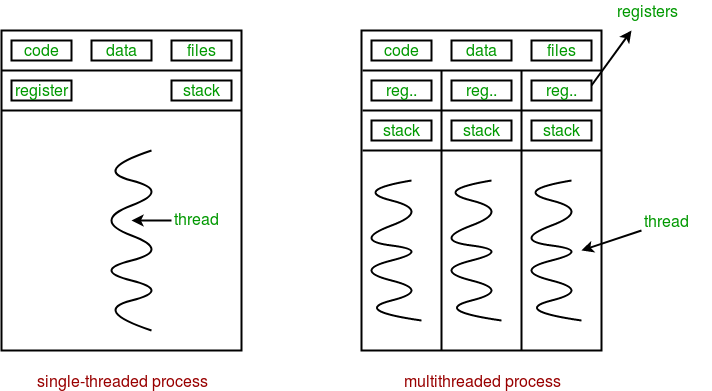Multithreading is defined as the ability of a processor to execute multiple threads concurrently.

In a simple, single-core CPU, it is achieved using frequent switching between threads. This is termed as context switching. In context switching, the state of a thread is saved and state of another thread is loaded whenever any interrupt (due to I/O or manually set) takes place. Context switching takes place so frequently that all the threads appear to be running parallely (this is termed as multitasking).

Consider the diagram below in which a process contains two active threads: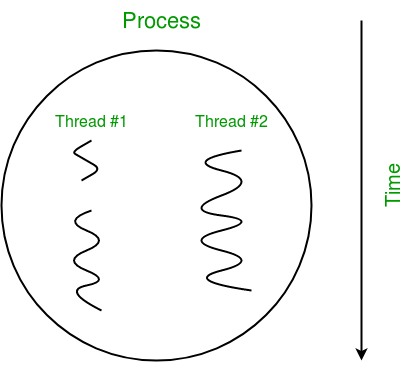Multithreading in Python

In Python, the threading module provides a very simple and intuitive API for spawning multiple threads in a program.

Let us consider a simple example using threading module:

 `# Python program to illustrate the concept``# of threading``# importing the threading module``import` `threading`` ` `def` `print_cube(num):``    ``"""``    ``function to print cube of given num``    ``"""``    ``print``(``"Cube: {}"``.``format``(num ``*` `num ``*` `num))`` ` `def` `print_square(num):``    ``"""``    ``function to print square of given num``    ``"""``    ``print``(``"Square: {}"``.``format``(num ``*` `num))`` ` `if` `__name__ ``=``=` `"__main__"``:``    ``# creating thread``    ``t1 ``=` `threading.Thread(target``=``print_square, args``=``(``10``,))``    ``t2 ``=` `threading.Thread(target``=``print_cube, args``=``(``10``,))`` ` `    ``# starting thread 1``    ``t1.start()``    ``# starting thread 2``    ``t2.start()`` ` `    ``# wait until thread 1 is completely executed``    ``t1.join()``    ``# wait until thread 2 is completely executed``    ``t2.join()`` ` `    ``# both threads completely executed``    ``print``(``"Done!"``)`
```Square: 100
Cube: 1000
Done!
```

Let us try to understand the above code:

• To import the threading module, we do:
```import threading
```
• To create a new thread, we create an object of Thread class. It takes following arguments:
• target: the function to be executed by thread
• args: the arguments to be passed to the target function

In above example, we created 2 threads with different target functions:

```t1 = threading.Thread(target=print_square, args=(10,))
t2 = threading.Thread(target=print_cube, args=(10,))
```
• To start a thread, we use start method of Thread class.
```t1.start()
t2.start()
```
• Once the threads start, the current program (you can think of it like a main thread) also keeps on executing. In order to stop execution of current program until a thread is complete, we use join method.
```t1.join()
t2.join()
```

As a result, the current program will first wait for the completion of t1 and then t2. Once, they are finished, the remaining statements of current program are executed.

Consider the diagram below for a better understanding of how above program works: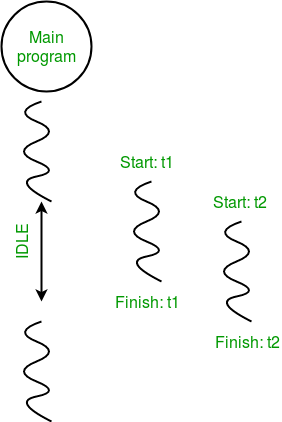Consider the python program given below in which we print thread name and corresponding process for each task:

 `# Python program to illustrate the concept``# of threading``import` `threading``import` `os`` ` `def` `task1():``    ``print``(``"Task 1 assigned to thread: {}"``.``format``(threading.current_thread().name))``    ``print``(``"ID of process running task 1: {}"``.``format``(os.getpid()))`` ` `def` `task2():``    ``print``(``"Task 2 assigned to thread: {}"``.``format``(threading.current_thread().name))``    ``print``(``"ID of process running task 2: {}"``.``format``(os.getpid()))`` ` `if` `__name__ ``=``=` `"__main__"``:`` ` `    ``# print ID of current process``    ``print``(``"ID of process running main program: {}"``.``format``(os.getpid()))`` ` `    ``# print name of main thread``    ``print``(``"Main thread name: {}"``.``format``(threading.current_thread().name))`` ` `    ``# creating threads``    ``t1 ``=` `threading.Thread(target``=``task1, name``=``'t1'``)``    ``t2 ``=` `threading.Thread(target``=``task2, name``=``'t2'``)  `` ` `    ``# starting threads``    ``t1.start()``    ``t2.start()`` ` `    ``# wait until all threads finish``    ``t1.join()``    ``t2.join()`
```ID of process running main program: 11758
Main thread name: MainThread
Task 1 assigned to thread: t1
ID of process running task 1: 11758
Task 2 assigned to thread: t2
ID of process running task 2: 11758
```

Let us try to understand the above code:

• We use os.getpid() function to get ID of current process.
```print("ID of process running main program: {}".format(os.getpid()))
```

As it is clear from the output, the process ID remains same for all threads.

• We use threading.main_thread() function to get the main thread object. In normal conditions, the main thread is the thread from which the Python interpreter was started. name attribute of thread object is used to get the name of thread.
```print("Main thread name: {}".format(threading.main_thread().name))
```
• We use the threading.current_thread() function to get the current thread object.
```print("Task 1 assigned to thread: {}".format(threading.current_thread().name))
```

The diagram given below clears the above concept: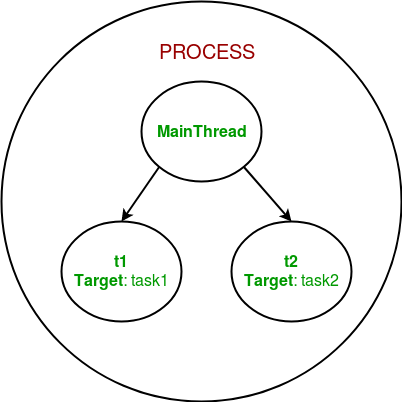So, this was a brief introduction to multithreading in Python. The next article in this series covers synchronization between multiple threads.

Multithreading in Python | Set 2 (Synchronization)

This article is contributed by Nikhil Kumar. If you like GeeksforGeeks and would like to contribute, you can also write an article using contribute.geeksforgeeks.org or mail your article to contribute@geeksforgeeks.org. See your article appearing on the GeeksforGeeks main page and help other Geeks.

Please write comments if you find anything incorrect, or you want to share more information about the topic discussed above.

Attention geek! Strengthen your foundations with the Python Programming Foundation Course and learn the basics.

To begin with, your interview preparations Enhance your Data Structures concepts with the Python DS Course.

My Personal Notes arrow_drop_up